Question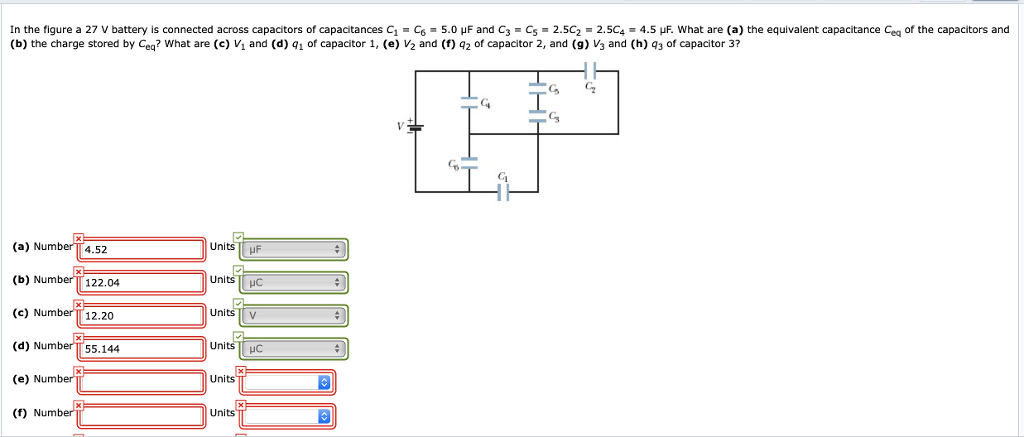a)

C3 and C5 are in series and their combination is given as

C35 = C3 C5/(C3 + C5) = (4.5) (4.5)/(4.5 + 4.5) = 2.25 uF

C2 , C4 and C35 are in parallel and their combination is given as

C2345 = C2 + C4 + C35 = 1.8 + 1.8 + 2.25 = 5.85 uF

C1 and C6 are in parallel and their combination is given as

C16 = C1 + C6 = 5 + 5 = 10 uF

C16 and C2345 are in series and their combination is given as

Ceq = C16 C2345 /(C16 + C2345 )

Ceq = (10) (5.85) /(10 + 5.85)

Ceq = 3.7 uF

b)

Q = charge stored by the combination of capacitors

V = battery Voltage = 27 Volts

Charge stored is given as

Q = Ceq V

Q = (3.7) (27)

Q = 99.9 uC

c)

V1 = Voltage across C1 = V16 = Voltage across the combination of C1 and C6 (Since in parallel voltage remain the same)

Q1 = charge in C1 = Q16 = charge in C16 = Q = 99.9 uC                  (Since charge remain same in series)

Using the equation

Q1 = C1 V1

99.99 = (5) V1

V1 = 19.98 Volts

d)

Q1 = charge in C1 = Q16 = charge in C16 = Q = 99.9 uC

e)

V2 = Voltage across C2 = Voltage across the parallel combination C2345 = V - V16 = 27 - 19.98 = 7.02 Volts   (Since in parallel voltage remain the same)

f)

Q2 = charge in C2 = ?

Using the equation

Q2 = C2 V2

Q2 =(1.8) (7.02)

Q2 = 12.6 uC

#### Earn Coins

Coins can be redeemed for fabulous gifts.

Similar Homework Help Questions
• ### In the figure a 27 V battery is connected across capacitors of capacitances C1-C6-3.0 μF and...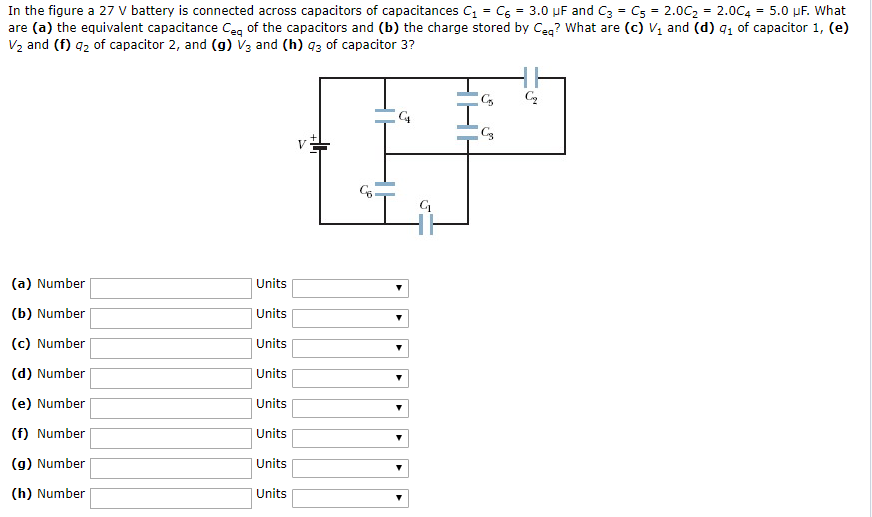In the figure a 27 V battery is connected across capacitors of capacitances C1-C6-3.0 μF and C3-Cs* 2.0C,-2.0C4-5.0 μF, what are (a) the equivalent capacitance Ceq of the capacitors and (b) the charge stored by Ceq? What are (c) V1 and (d) q1 of capacitor 1, (e) V2 and (f) 2 of capacitor 2, and (g) V3 and (h) 3 of capacitor 3? Gs C2 C4 CS C5 (a) Number (b) Number (c) Number (d) Number (e) Number (f) Number...

• ### In the figure a 23 V battery is connected across capacitors of capacitances C1 = C6 = 5.0 μF and C3 = C5=...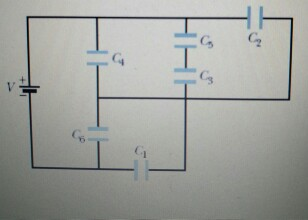In the figure a 23 V battery is connected across capacitors of capacitances C1 = C6 = 5.0 μF and C3 = C5= 2.0C2 = 2.0C4 = 5.0 μF. What are (a) the equivalent capacitance Ceq of the capacitors and (b) the charge stored by Ceq? What are (c) V1 and (d) q1 of capacitor 1,(e) V2 and (f) q2 of capacitor 2, and (g) V3 and (h) q3 of capacitor 3?

• ### In the figure a 27 V battery is connected across capacitors of capacitances C1 = C6...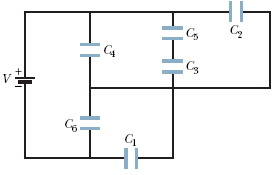In the figure a 27 V battery is connected across capacitors of capacitances C1 = C6 = 5.0 μF and C3 = C5 = 1.5C2 = 1.5C4 = 6.0 μF. What are (a) the equivalent capacitance Ceq of the capacitors and (b) the charge stored by Ceq? What are (c) V1 and (d) q1 of capacitor 1, (e) V2 and (f) q2 of capacitor 2, and (g) V3 and (h) q3 of capacitor 3? C HHH

• ### In the figure a 15 V battery is connected across capacitors of capacitances C1-Cg = 3.0...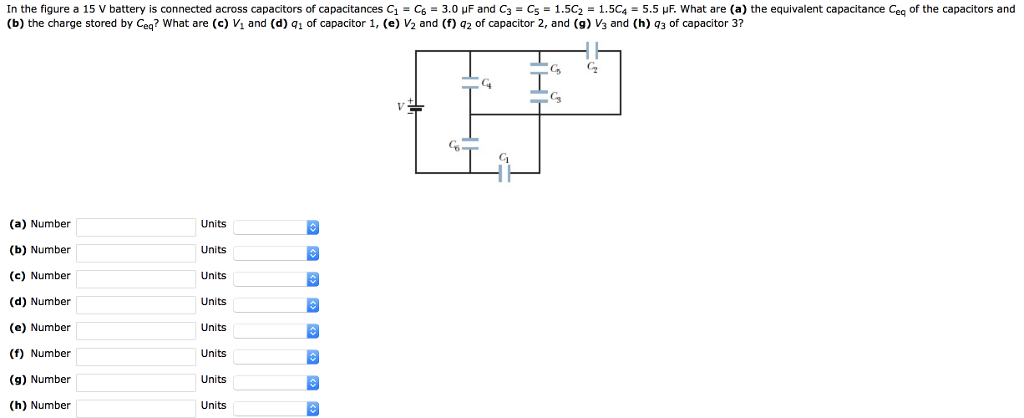In the figure a 15 V battery is connected across capacitors of capacitances C1-Cg = 3.0 μF and C3-C5-1.5C2-1.5C4-5.5岠what are (a) the equivalent capacitance Ceq of the capacitors and (b) the charge stored by Ceq? What are (c) Vi and (d) qi of capacitor 1, (e) V2 and (f) q2 of capacitor 2, and (9) V3 and (h) q3 of capacitor 3? G C C3 G6 (a) Number (b) Number (c) Number (d) Number (e) Number (f) Number (g) Number...

• ### In the figure a 15 V battery is connected across capacitors of capacitances C1-Cg = 3.0...In the figure a 15 V battery is connected across capacitors of capacitances C1-Cg = 3.0 μF and C3-C5-1.5C2-1.5C4-5.5岠what are (a) the equivalent capacitance Ceq of the capacitors and (b) the charge stored by Ceq? What are (c) Vi and (d) qi of capacitor 1, (e) V2 and (f) q2 of capacitor 2, and (9) V3 and (h) q3 of capacitor 3? G C C3 G6 (a) Number (b) Number (c) Number (d) Number (e) Number (f) Number (g) Number...

• ### Potential across a CapacitorIn Fig. 25-31 a20.0 V battery is connected across capacitors of capacitances C1 = C6 = 2.9 μF and C3 = C5 = 2.00C2 = 2.00C4 = 4.7 μF.Fig. 25-31http://i.imgur.com/IudoK.jpgWhat are(a) the equivalent capacitance Ceq of the capacitorsCeq = 3.18(b) the charge stored by Ceq?q = 63.6What are(c) V1 and (d) q1 of capacitor 1,V1 =q1 = (e) V2(f) q2 of capacitor 2,V2 = V q2 = (g) V3 and (h) q3 of capacitor 3,V3 =q3 =

• ### Question 7 Your answer is partially correct. Try again. In the figure a 19 V battery...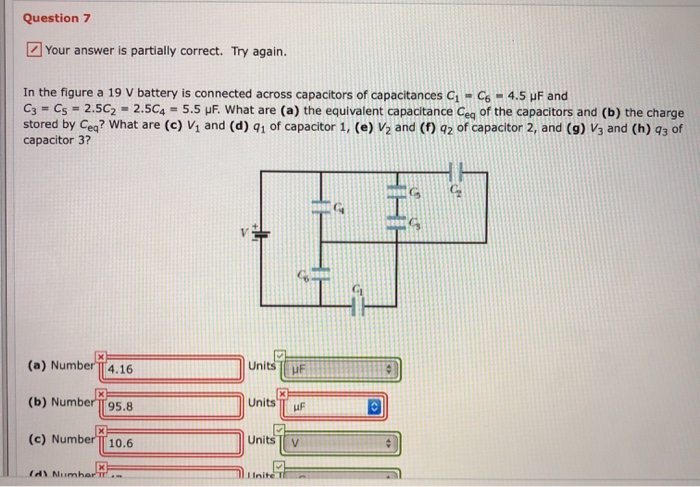Question 7 Your answer is partially correct. Try again. In the figure a 19 V battery is connected across capacitors of capacitances C1-C6 - 4.5 pF and Cz = C5 = 2.5C2 - 2.5C4 - 5.5 UF. What are (a) the equivalent capacitance Cea of the capacitors and (b) the charge stored by Ceg? What are (c) Vand (d) 41 of capacitor 1, (e) V2 and (1) 92 of capacitor 2, and (9) V3 and (h) 93 of capacitor 3?...

• ### in the ngure a s v battery is connected across capacitors of capacitances c.-cs = 4.0...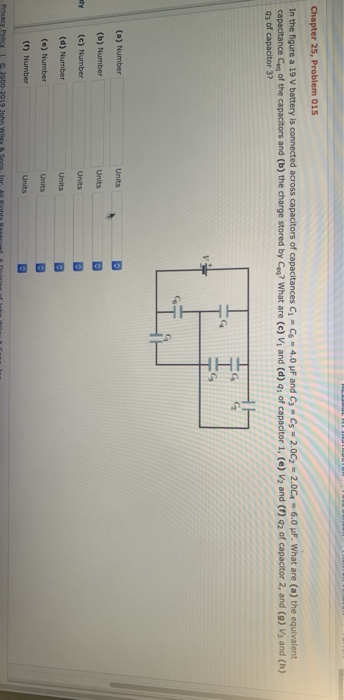in the ngure a s v battery is connected across capacitors of capacitances c.-cs = 4.0 μF and C3-C5 = 2.0C2-2004-6.0 μF. What are (a) the equivalent capacitance of the capacitors and (b) the charge stored by ㎝? what are (c) vi and (d) q1 of capacitor 1, (e) V2 and (f) q2 of capacitor 2, and (g) Vg and (h) 43 of capacitor 3? (a) Number (b) Number dy (c) Number (d) Number (e) Number (1) Number (a) Number...

• ### Charge on Capacitor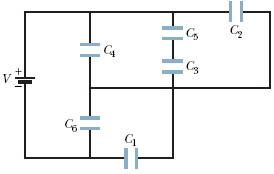In Fig. 25-31 a25 V battery is connected across capacitors of capacitances C1 = C6 = 3.5 μF and C3 = C5 = 2.5C2 = 2.5C4 = 5.5 μF. What are the(a) potential V3 across and(b) charge q3 (in C) on capacitor 3?

• ### please help. I do not understand Question 6 Your answer is partially correct. Try again. In...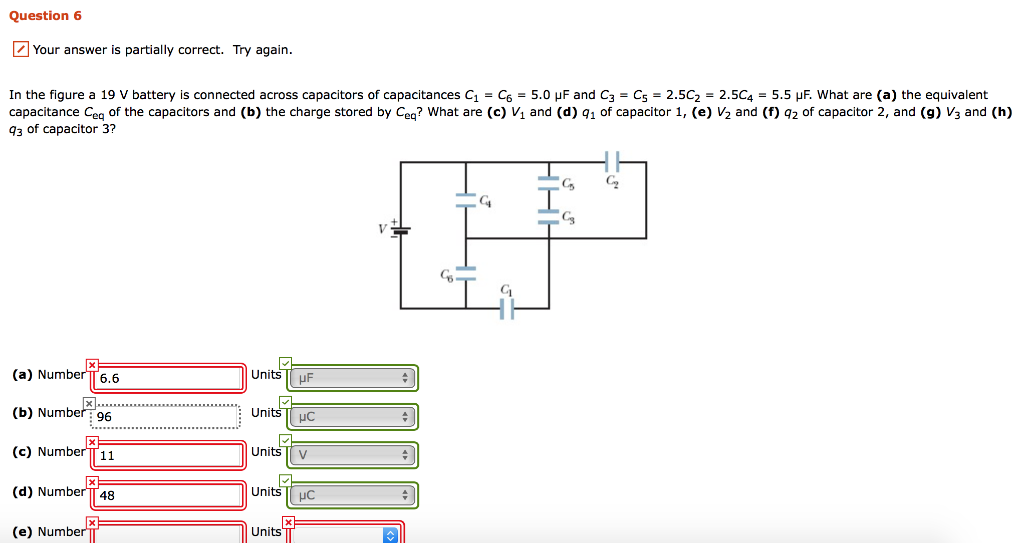please help. I do not understand Question 6 Your answer is partially correct. Try again. In the figure a 19 V battery is connected across capacitors of capacitances C1 = C6 = 5.0 pF and C3 = C5 = 2.5C2 = 2.5C4 = 5.5 UF. What are (a) the equivalent capacitance Cea of the capacitors and (b) the charge stored by Ceg? What are (c) V1 and (d) 41 of capacitor 1, (e) V2 and (f) 42 of capacitor 2,...